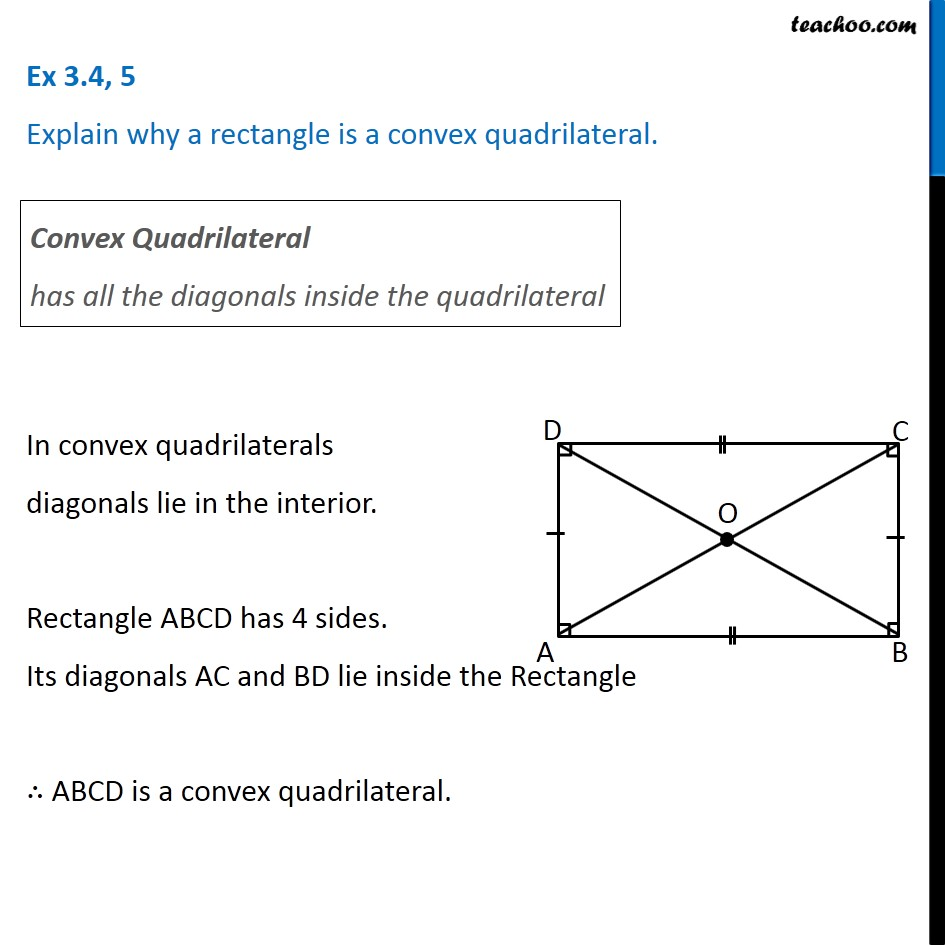1. Chapter 3 Class 8 Understanding Quadrilaterals
2. Serial order wise
3. Ex 3.4

Transcript

Ex 3.4, 5 Explain why a rectangle is a convex quadrilateral. Convex Quadrilateral has all the diagonals inside the quadrilateral In convex quadrilaterals diagonals lie in the interior. Rectangle ABCD has 4 sides. Its diagonals AC and BD lie inside the Rectangle ∴ ABCD is a convex quadrilateral.

Ex 3.4